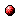DOC HOME SITE MAP MAN PAGES GNU INFO SEARCH

# (guile-tut.info.gz) Jump StartInfo Catalog(guile-tut.info.gz) Top(guile-tut.info.gz) Top(guile-tut.info.gz) Introduction
```
1 Jump Start
************

Before giving an overview of Guile, I present some simple commands and
programs that you can type to get going immediately.

Start by invoking the Guile interpreter.  Usually you do this by just
typing `guile'.  Then type (or paste) the following expressions at the
prompt; the interpreter's response is preceded (in this manual) by =>.

<shell-prompt> guile

(+ 20 35)
=> 55
(define (recursive-factorial n)
(if (zero? n)
1
(* n (recursive-factorial (- n 1)))))
(recursive-factorial 5)
=> 120
(quit)

In this example we did some simple arithmetic `(+ 20 35)' and got the
answer `55'.  Then we coded the classic (and rather wasteful) factorial
algorithm and computed the factorial of `55'.  Finally we quit with
`(quit)'.

We can find out about some of Scheme's nice features by asking for the
factorial of some big number, say `500'.  On some systems the correct
answer will be returned (I do not indicate calling and leaving the
guile session anymore).

(recursive-factorial 500)
=> 1220136825991110068701238785423046926253574342803192842192413588
3858453731538819976054964475022032818630136164771482035841633787
2207817720048078520515932928547790757193933060377296085908627042
9174547882424912726344305670173270769461062802310452644218878789
4657547771498634943677810376442740338273653974713864778784954384
8959553753799042324106127132698432774571554630997720278101456108
1188373709531016356324432987029563896628911658974769572087926928
8712817800702651745077684107196243903943225364226052349458501299
1857150124870696156814162535905669342381300885624924689156412677
5654481886506593847951775360894005745238940335798476363944905313
0623237490664450488246650759467358620746379251842004593696929810
2226397195259719094521782333175693458150855233282076282002340262
6907898342451712006207714640979456116127629145951237229913340169
5523638509428855920187274337951730145863575708283557801587354327
6888868012039988238470215146760544540766353598417443048012893831
3896881639487469658817504506926365338175055478128640000000000000
0000000000000000000000000000000000000000000000000000000000000000
00000000000000000000000000000000000000000000000

The result is an example of Scheme's _bignumbers_.  However, there
are operating environments that provide (by default) too little stack
space.  They will instead produce an error message like this:

(recursive-factorial 500)
-|
ERROR: Stack overflow
ABORT: (stack-overflow)

Rather than enlarging the system's stack, we can implement the
algorithm such that it does not consume increasing stack space.  This
is called a _tail recursive_ implementation.  The following definition
is tail recursive and so should work on all systems.

(define (tail-recursive-factorial n)
(define (loop k l)
(if (zero? k) l
(loop (- k 1) (* k l))))
(loop n 1))

(tail-recursive-factorial 500)
=> 1220136825991110068701238785423046926253574342803192842192413588
;; ... skipped

This is the most basic use of Guile: a simple Scheme interpreter.  In
the rest of this tutorial I will show you how Guile has many facets: it
is also an _extensible_ interpreter (to which many features can be
easilly added) and an _embeddable_ interpreter (which can be invokedInfo Catalog(guile-tut.info.gz) Top(guile-tut.info.gz) Top(guile-tut.info.gz) Introduction